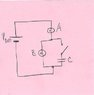# Conceptual RC Circuit questions

• DrummingAtom
In summary, the current through the components is not equal, and the voltage across each component is different.f

## Homework Statement

After a long time after the switch is closed.

1) Rank the current of each component.
2) What is the voltage across each component?

## The Attempt at a Solution

1) The current across B is the same as the capacitor C because the capacitor is fully charged. There is no current across Vbattery and A because the capacitor now has positive charges on the top and negative charges on the bottom so there is no potential outside the parallel part (B and C).

2) 0 V for A and Vbattery. But B and C have the total voltage across each.

Is this right?

I'm thinking that the parallel part creates it's own self powered loop and blocks out the rest, the battery and A.

Thanks for any help.

#### Attachments

•RC Circuit.jpg
25.5 KB · Views: 448
Current flows THROUGH. Voltage appears ACROSS.

After a long time the capacitor voltage settles to whatever the circuit around it dictates. In this case it won't be equal to the battery voltage because the lamps are creating a voltage divider. To see this, use the rule of thumb that says that at steady state the capacitor current will be zero and its voltage fixed, so for all intents and purposes you can simply ignore it (remove it from the circuit entirely). What does the remaining circuit look like?

## Homework Statement

After a long time after the switch is closed.

1) Rank the current of each component.
2) What is the voltage across each component?

## The Attempt at a Solution

1) The current across B is the same as the capacitor C because the capacitor is fully charged. There is no current across Vbattery and A because the capacitor now has positive charges on the top and negative charges on the bottom so there is no potential outside the parallel part (B and C).
Sorry, this is totally wrong.
The capacitor is parallel connected to B. Why should the same current flow through both? What is the same across them?
The voltage of the battery is given, Vbat. Is there a closed path between the terminals through A and B? Can current flow from the positive pole of the battery to the negative one?

2) 0 V for A and Vbattery. But B and C have the total voltage across each.

Is this right?

No. The voltage of the battery is constant, Vbat.

ehild